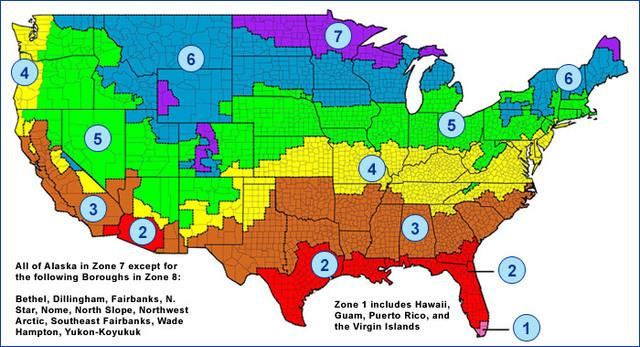Mar 31, 2021

# This Is What R-Value Is and Why It’s Important

Tuesday, May 19th, 2020

If you’re considering ways to improve your home’s energy efficiency, you’ve probably come upon the term “R-value.” Well, what does “R-value” actually mean? We have you covered.

## What is R-value?

Simply put, the R in insulation R-value indicates the resistance of heat transfer through the insulation.

The higher the R-value, the greater the thermal performance. The R-value depends on the type of insulation, its thickness, and its density. The more resistant, the longer it takes for heat to flow out of the room through the insulation.

## How is R-value Calculated?

R-value is calculated by using the following formula:

R-Value= (Temperature Difference x Area x Time) / Heat Loss

• Temperature difference = the difference in temperature in degrees Fahrenheit between the outside and the interior room
• Area = the square footage of the room that stands between the inside and the outside
• Time = time elapsed in hours
• Heat loss = heat loss, expressed in BTUs

R-value is calculated per inch of width.

For example, blown fiberglass installed in the attic has an R-value of 2.2 per inch, so 12 inches of it would give you an R-value of 26.4 in your attic installation project.

The R-value of insulation is important. One factor to consider is that it doesn’t matter how high your insulation’s R-value is if cold air is leaking through cracks and gaps. This can lessen the effectiveness of your insulation. Therefore, it is best practice to seal any cracks and gaps before insulating the space.

Another factor to consider is the environment you live in. Naturally, the colder your climate, the more insulation you’ll need. Here in Wisconsin, we live in zone 5 to 8. Here is a map of climate zones and R-value chart by zone.(Source: Energystar.gov)